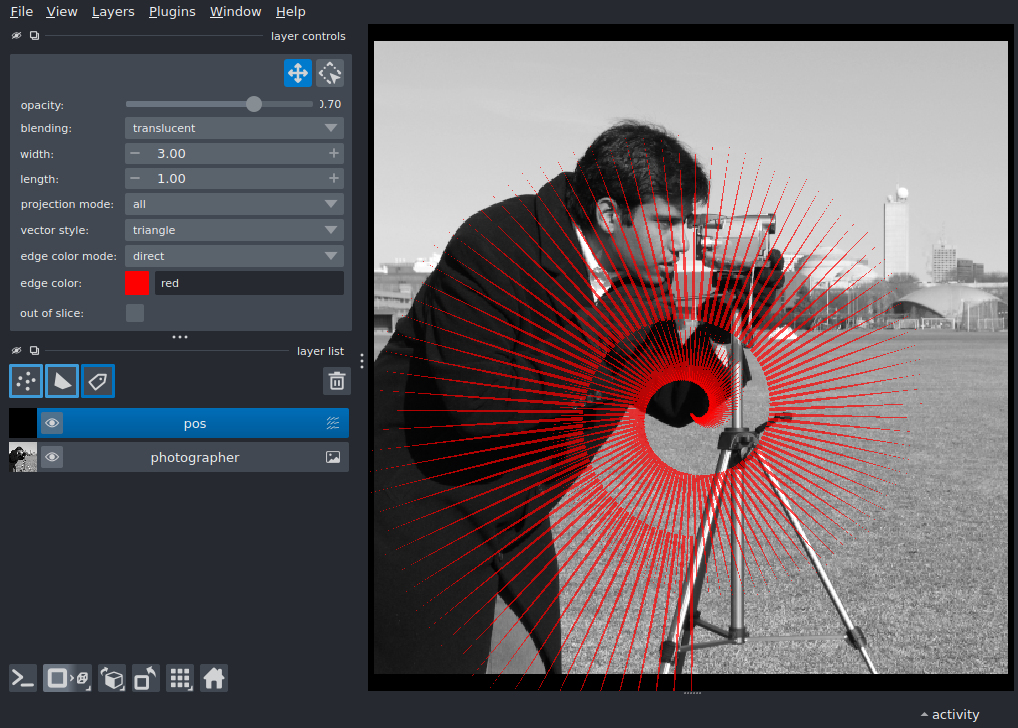This example generates an image of vectors Vector data is an array of shape (N, 4) Each vector position is defined by an (x, y, x-proj, y-proj) element where * x and y are the center points * x-proj and y-proj are the vector projections at each center

Tags: visualization-basic```import napari
from skimage import data
import numpy as np

# create the viewer and window
viewer = napari.Viewer()

# sample vector coord-like data
n = 200
pos = np.zeros((n, 2, 2), dtype=np.float32)
phi_space = np.linspace(0, 4 * np.pi, n)

# assign x-y position
pos[:, 0, 0] = radius_space * np.cos(phi_space) + 300
pos[:, 0, 1] = radius_space * np.sin(phi_space) + 256

# assign x-y projection
pos[:, 1, 0] = 2 * radius_space * np.cos(phi_space)
pos[:, 1, 1] = 2 * radius_space * np.sin(phi_space)